126
Created byMaster Student

Chemistry Practice Test #6

1 / 30

If the temperature of a gas is quadrupled from 40K to 160K, which of the following accurately describes the change in the average kinetic energy of the gas?

2 / 30

Parallax Error may occur when:

3 / 30

Which piece of laboratory glassware is best used to measure 1.000 L of solution accurately?

4 / 30

Chemical reactions can be classified as either heterogeneous or homogeneous. Which of the following equations below is best classified as a heterogeneous reaction?

5 / 30

What is the theoretical yield of iron when 2.00 grams of carbon is reacted with 26.0 grams of Fe2O3?

2 Fe2O3 + 3 C → 4 Fe + 3 CO2

6 / 30

What is the empirical formula of a compound that is 51.9% carbon, 4.86% hydrogen, and 43.2% bromine

7 / 30

If 7.000g of a solid displaced 21.0 mL of water when dropped into a graduated cylinder, what is the density of the solid?

8 / 30

What is the theoretical yield of ethyl ethanoate when 100 grams of ethanoic acid is reacted with 100 grams of ethyl alcohol?

CH3COOH + CH3CH2OH → CH3COOCH2CH3 + H2O

9 / 30

Which of the following molecules cannot hydrogen bond with molecules identical to itself but can hydrogen bond with one of the molecules above or below it in the following responses?

10 / 30

Iron(III) hydroxide has Ksp = 1.6 × 10-39. What is the molar solubility of this compound?

11 / 30

Balance the following equation:

Butane + O2 -> H2O + CO2

12 / 30

Methylamine, CH3NH2, has a Kb = 4.4 × 10-4. If a 0.0100 M solution of methylamine is prepared, the expected pH will be in which one of the following pH ranges?

13 / 30

The forces responsible for the relatively high melting point and boiling point of water, H2O, are:

14 / 30

A 25 g sample of a liquid was heated to 100 °C and then quickly transferred to an insulated container holding 100 g of water at 22 °C. The temperature of the mixture rose to reach a final temperature of 35 °C. Which of the following can be concluded?

15 / 30

Which of the reactions below will be thermodynamically favoured at all temperatures?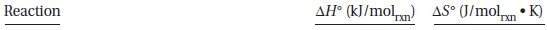• A.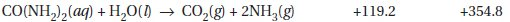• B.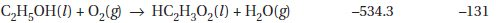• C.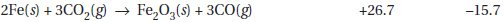• D.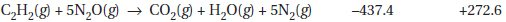16 / 30

Why do vinegar (a dilute solution of ethanoic acid in water) and vegetable oil (long-chain organic acids esterified with glycerol) not mix to form solutions?

17 / 30

According to the ideal gas law at STP, which of the following is the value of the ratio between moles (assume 1 mole), the gas constant R, temperature and the pressure

18 / 30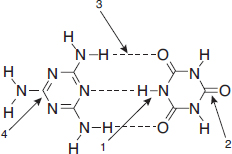In the diagram above, which labelled arrow is pointing toward a hydrogen bond?

19 / 30

The rate of a chemical reaction is determined by

20 / 30

From the solubility rules, which of the following is true?

21 / 30

S(s) + O2(g) → SO2(g)

The reaction of sulfur with oxygen is written in equation form above. This equation can be interpreted in all of the following ways except

22 / 30

Which of the following molecules is expected to have the highest normal boiling point?

23 / 30

Which of the following compounds would have the highest boiling point?

24 / 30

A 50.0 mL aliquot of a solution containing Al(OH)3 is titrated with 0.0500 molar H2SO4. The endpoint is reached when 35.0 mL of the sulfuric acid has been added. What is the molarity of the aluminum hydroxide solution?

A.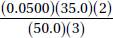B.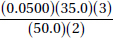C.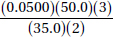D.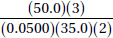25 / 30

An experiment was performed to determine the moles of hydrogen gas formed (collected over water) when an acid reacts with magnesium metal. To do this, a piece of dry magnesium was weighed. Then 50 mL of hydrogen was collected. Next, the Mg was dried to remove approximately 0.1 mL of water and weighed again to see how much Mg had reacted. The atmospheric pressure was measured as 755 torrs. The volume of hydrogen was measured and converted into moles of hydrogen. Which mistake will give the smallest error in the result?

26 / 30

Identify the Brønsted-Lowry conjugate acid-base pair in the following list.

27 / 30

The standard galvanic cell voltage, E°cell

28 / 30

NT explosions are very fast reactions involving the production of enormous quantities of heat and gaseous products. Thermodynamically, explosions are best described as:

29 / 30

How is the vapor pressure of a liquid affected if it is boiled in a pressure cooker?

30 / 30

Which is correct about the calcium atom?